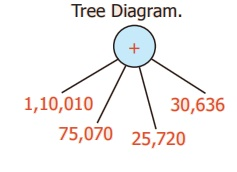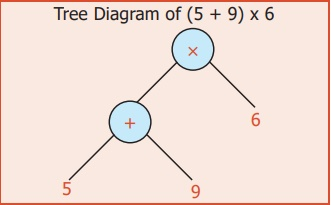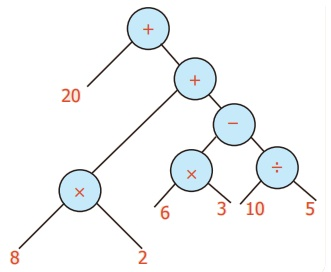Home | | Maths 6th Std | Information Processing

# Information Processing

Learning Objectives * To know how to represent numerical and algebraic expressions by tree diagrams. * To know how to write numerical and algebraic expressions from tree diagrams.

CHAPTER 5

INFORMATION PROCESSING

Learning Objectives

* To know how to represent numerical and algebraic expressions by tree diagrams.

* To know how to write numerical and algebraic expressions from tree diagrams.

Introduction

In today's digital era, it is almost impossible to imagine a day without computers. Right from small shops to big software companies, the use of computers is inevitable. If there are no computers, most of the works will be stopped. Computers are able to find solutions even for complicated numerical expression and algebraic expression in quick and easy way. The answer given by the computer will be very precise and need not to be recalculated. There will be a question, how the computer read these expression?.

Yes, Computers use Tree diagram to perform billions of operations in a uniform way and gives the answer. We will learn about the Tree diagram for both numeric and algebraic expressions in this chapter.

MATHEMATICS ALIVE – INFORMATION PROCESSING IN REAL LIFEConsider the numerical expression [(9 − 4 ) × 8 ] ÷ [ ( 8 + 2 ) × 3]. We can try to understand the expression in a better way through the tree diagram.

1) Let us consider e1 = (9 − 4) × 8, e2 = ( 8 + 2 ) × 3 we get2) e1= f1 × f2 Where, f1 = 9 − 4 and f2 = 83) f1 = r1 − r2 where r1 = 9 and r2 = 4. f1 is represented as:Similarly, the trees can be developed from e2.

4) Putting all together, we get the following tree diagramIt is a picture which look like an upside-down tree! Every node has one or two branches. And the leaves are numbers. The branching  nodes have operations on them. It is called tree diagram and the tree diagrams are general ways of representing arithmetical expressions. Here trees are drawn upside down.

The root is at the top, the leaves are at the bottom. Since all the arithmetical operations are binary (Involving two numbers) we have only 2 way branching in the tree.

Can you represent the addition of four numbers in the same way? Yes, there is a way for addition of 4 numbers.Let us learn how to represent the statement problems in tree diagram

Example 1:

In the flower exhibition conducted at Ooty for 4 days the number of tickets sold on the first, second, third and fourth days are 1,10,010; 75,070; 25,720 and 30,636 respectively. Find the total number of tickets sold.

Solution:Number of tickets sold on the first day = 1,10,010

Number of tickets sold on the second day = 75,070

Number of tickets sold on the third day = 25,720

Number of tickets sold on the fourth day = 30,636

Total = 2,41,436

Total number of tickets sold = 2,41,436

Example 2:

In one year, a paper company had sold 6,25,610 notebooks out of a stock of 7,50,800 notebooks. Find the number of notebooks left unsold.

Solution:

Tree Diagram.Number of Notebooks in stock     = 7,50,800

Number of Notebooks sold          = 6,25,610

Number of notebooks left unsold = 1,25,190

Example 3:

Vani and Kala along with three other friends went to a butter milk shop.

The cost of one butter milk is ₹ 6. If 9 more friends joined them, then how much money did they have to pay? Vani said they had to pay ₹ 84 whereas Kala said they had to pay ₹ 59. Who is correct ?

Solution:

Tree Diagram of (5 + 9) x 6This confusion can be resolved by using the brackets in the correct places like (5+9) × 6.

It is further clear from the tree diagram.

Therefore Vani is correct

Example 4:

If a ration shop has distributed 1,00,000 kg of rice to 5000 families, then find the quantity of rice given to each family?

Solution:

Tree Diagram.Quantity of rice to be distributed to 5000 = 1,00,000 kg families

Quantity of rice distributed to each family = 1,00,000 ÷ 5,000

= 20 kg

Each family was given 20 kg of rice.

Example 5:

Convert into a Tree diagram (9 × 5) + (10 × 12)

Solution:Example 6:

Convert into a Tree diagram (10 × 9) − (8 × 2) + 3

Solution:Example 7:

Convert into a Tree diagram [ 8+ (5 × 2)] – [(2 × 3) + 5]

Solution:Example 8:

Convert into a Tree diagram [(9−4) × 8] + [(8+2) × 3]

Solution:Example 9 :

Convert into a Tree diagram {[ (10 × 5) + 6] × [5 + (6 − 2)]} ÷ [8 × (4 + 2)] .

Solution:Example 10:

Convert into a Tree diagram 20+ [8 × 2+ {(6 × 3) – 10 ÷ 5} ].

Solution:Tags : Term 2 Chapter 5 | 6th Maths , 6th Maths : Term 2 Unit 5 : Information Processing
Study Material, Lecturing Notes, Assignment, Reference, Wiki description explanation, brief detail
6th Maths : Term 2 Unit 5 : Information Processing : Information Processing | Term 2 Chapter 5 | 6th Maths